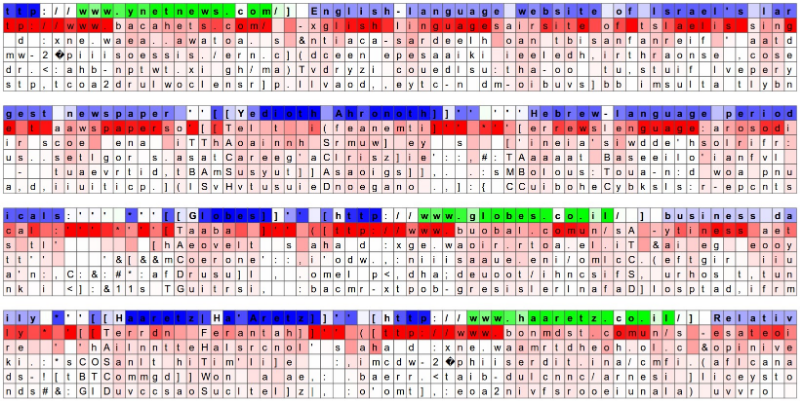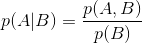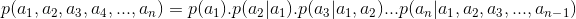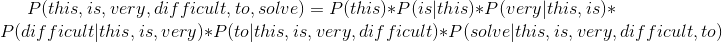# Language Model for NLPimg-1 : source : karpathy’s blog post

If you are interested in Natural Language Processing then Language Models are very crucial and important part of NLP that you need to understand.

Before the advancement of machine learning, specifically deep learning, language models were built based on different statistical approaches like : the n-gram based language model, hidden markov model, rule based NLP etc.

Language models are so useful now a days that most of the big tech companies and their highly valuable products are using it extensively. Google Assistant , Apple Siri, Microsoft Cortana, Amazon Alexa all are using language models in different purposes.

Here in this blog-post I will cover the two types of language models which will be used for auto completion:
1. Statistical Language Model : We will design an n-gram (tri-gram) based language model using the python’s nltk library and its own Reuters corpus.
2. Neural Language Model : Deep neural networks are the state of the art techniques and can be used in language model design. We will design a simple LSTM model which can be used for the same.

Contents:
1. Language Model Overview
2. N-gram based Language Model
3. Neural Language Model

# Language Model Overview

The main concept behind the language model is to predict the probability of a sequence of words or characters. All the NLP related applications like machine translation, POS tagging, speech recognition etc uses this concept of maintaining the sequence of words or characters.

So Language models try to get the relative likelihood of a word or character given a sequence of words or characters.

## Little Math (Conditional Probability and Chain rule)img-2 : Conditional probability formulaimg-3 : Chain rule

We use the chain rule to find the joint probability of the next word/character given the sequence of words/characters.img-4 : practical example

# N-gram based Language Model

An n-gram is a sequence of n consecutive tokens or words in a corpus.

For an example if we have a sentence like : medium is a good place for machine learning blogs
If we represent the above sentence using one-gram technique it will be something like this : [medium, is, a, good, place, for, machine, learning, blogs]
If we represent the above sentence using bi-gram technique it will be something like this : [medium is, is a, a good, good place, place for, for machine, machine learning, learning blogs]
If we represent the above sentence using tri-gram technique it will be something like this : [medium is a, is a good, a good place, good place for, place for machine, for machine learning, machine learning blogs]

Like this way it goes on.

We will calculate the probability by applying the chain rule (img-3 & img-4).
As I have mentioned chain rule calculates the joint probability of a sequence by using the conditional probability of a word given the previous words.
But calculating all the conditional probabilities is very complex and don’t have access to them.
In such a case we approximate the context of the word (an) by looking at the last word of context (an-1).
p(an|a1,a2,a3,a4,…,an-1) = p(an|an-1).

## Building n-gram model using python

As of now we have the basic understanding of how an n-gram model works and calculates the probability, we will build the same in python.
We are going to use the python’s nltk library and it’s Reuters corpus for the same.
Let’s import the dependencies first.

Now we will create our tri-gram model as a python nested dictionary structure where the key of the outer dictionary will be the two previous tokens (words) and the value will be another dictionary in which the key will be the third token (word) and its count.
Following is an example of the same.

`# two history/previous tokens as the key{('this', 'is'):{'very':2, # third token with out 2                 'nice':3, # third token with out 3                 'a':1}}   # third token with out 1`

Once the above model (nested dictionary) is created, we will calculate the percentage count of each third tokens with respect to the previous two tokens.
It will help us to predict the output properly with a probability measure.

So it works, like an auto completion technique we often see in our mobile devices.
Still there are few limitations are there of these kind of language models.

## Limitations of n-gram approach

1. Typically if we increase the ’n’ the model will try to perform better, but eventually we will need more computation power and huge resource of memory (RAM) for the same.
2. Here in this approach we are building the model based on the probability of words co-occurring. It will give zero probability to all the words that are not present in the training corpus, which is not at all desirable.

# Neural Language Model

Due to the advancement of Deep Neural Networks specifically Recurrent Neural Networks & more specifically LSTM (LONG SHORT TERM MEMORY), neural language models are becoming very powerful and state of the art techniques.

Here in this section we will create the same auto completion language model which will be based on sequence of characters.
For the data-set I have created one file which I have used for the training and validation purpose of the model. (I will provide both the source code and data-set GitHub link in the later section).

## Design Neural Language Model in Python

Before you jump into the code please have a look into these following blogs by Christopher Olah and Andrej Karpathy on LSTM and RNN.
These blogs are tremendous and I personally prefer them to read anytime I want.
We will create a language model which will take a sequence of characters instead of words and try to predict next characters.

Let’s import necessary modules

As the text has many unnecessary characters, punctuation, numbers, special characters etc. We need to clean them up for our model to predict only necessary things.

As part of pre-processing the cleaned text we will do the following steps before fitting the model.
1. We will now create a list of character sequences of fixed length for the training of the language model.
2. Encoding each character by means of assigning each character an unique number.
3. Split the data into training set and validation set where X will be the first n-1 characters and Y will be the nth character.

Now we are ready to fir our LSTM model.
NOTE : as part of building lstm model i have used two layered lstm networks and due to less computation resources I used very few data for the same. As part your activity you can play with the model by increasing or decreasing the layers, by changing the dropout rates, by changing the number of neurons in each layer, to get the best performance.

Once the model is trained we can test them to check the result.

You can change the input text slightly and can see the sensitivity of the model.
Also you can notice that the sequence doesn’t belong to the original corpus.

## Code Repo

You can get the data-set and entire ipython notebook here in GitHub.

## More from sourajit roy chowdhury

Machine learning Engineer